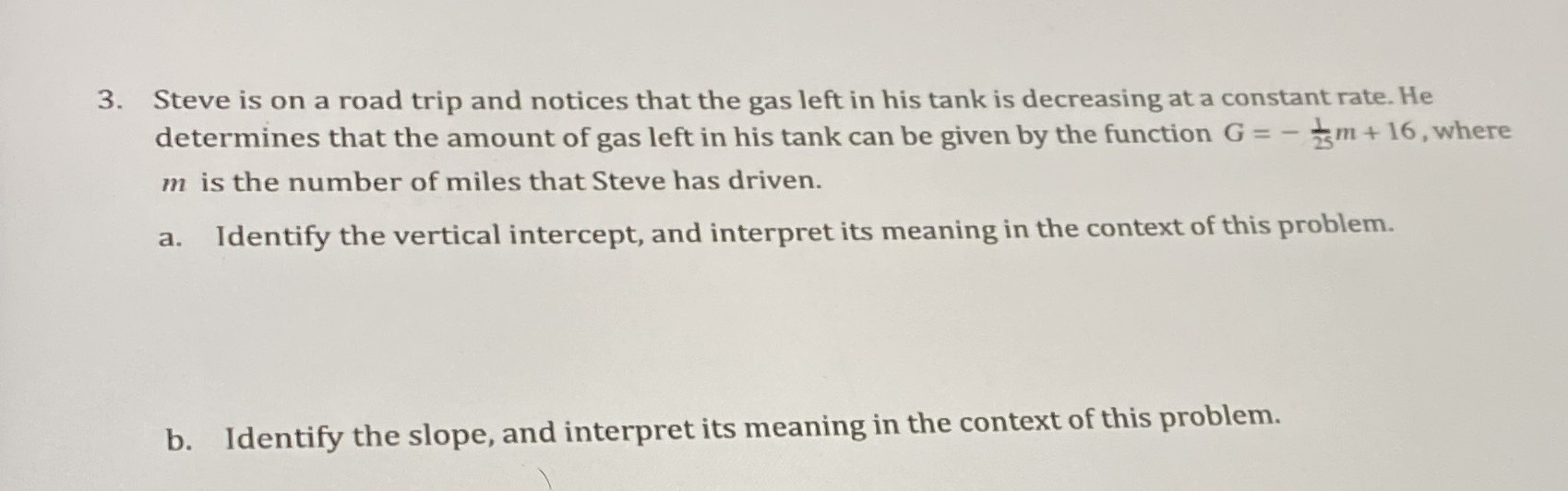### ¿Todavía tienes preguntas de matemáticas?

Pregunte a nuestros tutores expertos
Algebra
Pregunta3. Steve is on a road trip and notices that the gas left in his tank is decreasing at a constant rate. He determines that the amount of gas left in his tank can be given by the function$$G = - \frac { 1 } { 25 } m + 16$$ , where $$m$$ is the number of miles that Steve has driven.

a. Identify the vertical intercept, and interpret its meaning in the context of this problem.

b. Identify the slope, and interpret its meaning in the context of this problem.